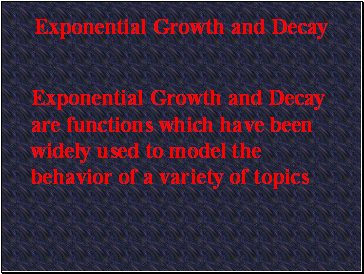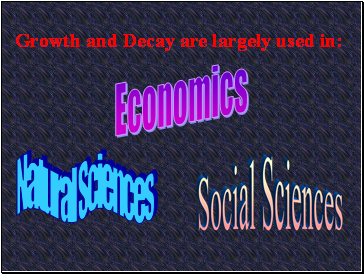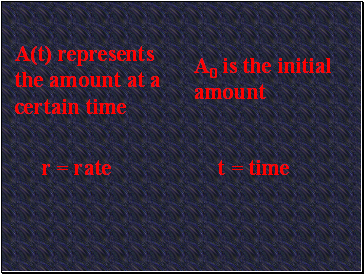# Growth & decayPage 1

#### WATCH ALL SLIDES

Slide 1## Exponential Growth and Decay

Exponential Growth and Decay are functions which have been widely used to model the behavior of a variety of topics

Slide 2## Growth and Decay are largely used in:

Economics

Natural Sciences

Social Sciences

Slide 3Growth can be modeled by the function:

While Decay can be modeled by:

Slide 4A(t) represents the amount at a certain time

A0 is the initial amount

r = rate

t = time